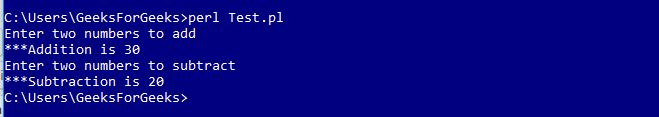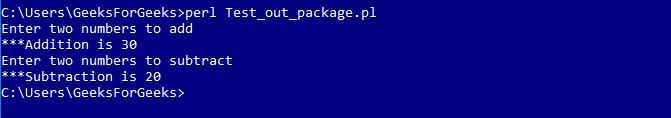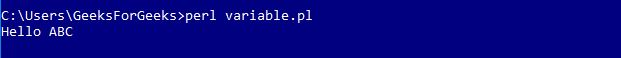# Packages in Perl

A Perl package is a collection of code which resides in its own namespace. Perl module is a package defined in a file having the same name as that of the package and having extension .pm. Two different modules may contain a variable or a function of the same name. Any variable which is not contained in any package belongs to the main package. Therefore, all the variables being used, belong to the ‘main’ package. With the declaration of additional packages, it is maintained that variables in different packages do not interfere with each other.

Declaration of a Perl Module

Name of the module must be the same as that of the package name and has a .pm extension.

Example : Calculator.pm

 `package` `Calculator; ` ` `  `# Defining sub-routine for Addition ` `sub` `addition ` `{ ` `    ``# Initializing Variables a & b ` `    ``\$a` `= ``\$_``; ` `    ``\$b` `= ``\$_``; ` `     `  `    ``# Performing the operation ` `    ``\$a` `= ``\$a` `+ ``\$b``; ` `     `  `    ``# Function to print the Sum ` `    ``print` `"\n***Addition is \$a"``; ` `} ` ` `  `# Defining sub-routine for Subtraction ` `sub` `subtraction ` `{ ` `    ``# Initializing Variables a & b ` `    ``\$a` `= ``\$_``; ` `    ``\$b` `= ``\$_``; ` `     `  `    ``# Performing the operation ` `    ``\$a` `= ``\$a` `- ``\$b``; ` `     `  `    ``# Function to print the difference ` `    ``print` `"\n***Subtraction is \$a"``; ` `} ` `1; `

Here, the name of the file is “Calculator.pm” stored in the directory Calculator. Notice that 1; is written at the end of the code to return a true value to the interpreter. Perl accepts anything which is true instead of 1.

Using a Perl Module

To use this calculator module, we use require or use functions. To access a function or a variable from a module, :: is used. Here is an example demonstrating the same:

Examples: Test.pl

 `#!/usr/bin/perl ` ` `  `# Using the Package 'Calculator' ` `use` `Calculator; ` ` `  `print` `"Enter two numbers to add"``; ` ` `  `# Defining values to the variables ` `\$a` `= 10; ` `\$b` `= 20; ` ` `  `# Subroutine call ` `Calculator::addition(``\$a``, ``\$b``); ` ` `  `print` `"\nEnter two numbers to subtract"``; ` ` `  `# Defining values to the variables ` `\$a` `= 30; ` `\$b` `= 10; ` ` `  `# Subroutine call ` `Calculator::subtraction(``\$a``, ``\$b``); `

Output:Accessing a Package from a different directory

If a file accessing the package lies outside the directory then we use ‘::’ to tell the path of the module. For example, file using calculator module is outside the calculator package so we write Calculator :: Calculator to load the module, where the value on left of ‘::’ represents package name and value on the right of ‘::’ represents Perl module name. Let’s see an example to understand this:

Examples: Test_out_package.pl outside Calculator directory

 `#!/usr/bin/perl ` ` `  `use` `GFG::Calculator; ``# Directory_name::module_name ` ` `  `print` `"Enter two numbers to add"``; ` ` `  `# Defining values to the variables ` `\$a` `= 10; ` `\$b` `= 20; ` ` `  `# Subroutine call ` `Calculator::addition(``\$a``, ``\$b``); ` ` `  `print` `"\nEnter two numbers to subtract"``; ` ` `  `# Defining values to the variables ` `\$a` `= 30; ` `\$b` `= 10; ` ` `  `# Subroutine call ` `Calculator::subtraction(``\$a``, ``\$b``); `

Output:Using Variables from modules

Variables from different packages can be used by declaring them before using. Following example demonstrates this
Examples: Message.pm

 `#!/usr/bin/perl ` ` `  `package` `Message; ` ` `  `# Variable Creation ` `\$username``; ` ` `  `# Defining subroutine ` `sub` `Hello ` `{ ` `  ``print` `"Hello \$username\n"``; ` `} ` `1; `

Perl file to access the module is as below
Examples: variable.pl

 `#!/usr/bin/perl ` ` `  `# Using Message.pm package ` `use` `Message; ` ` `  `# Defining value to variable ` `\$Message::username` `= ``"ABC"``; ` ` `  `# Subroutine call ` `Message::Hello(); `

Output:Begin and End Block

BEGIN and END block is used when we want to run some part of the code at the beginning and some part of the code at an end. The codes written within BEGIN{…} are executed at the beginning of the script while the codes written within END{…} are executed at the end of the script. The program below demonstrates this:
Examples: begineg.pl

 `#!/usr/bin/perl ` ` `  `# Predefined BEGIN block ` `BEGIN ` `{ ` `    ``print` `"In the begin block\n"``; ` `} ` ` `  `# Predefined END block ` `END ` `{ ` `    ``print` `"In the end block\n"``; ` `} ` ` `  `print` `"Hello Perl;\n"``; `

Output:

```In the begin block
Hello Perl;
In the end block```

My Personal Notes arrow_drop_upCheck out this Author's contributed articles.

If you like GeeksforGeeks and would like to contribute, you can also write an article using contribute.geeksforgeeks.org or mail your article to contribute@geeksforgeeks.org. See your article appearing on the GeeksforGeeks main page and help other Geeks.

Please Improve this article if you find anything incorrect by clicking on the "Improve Article" button below.

Article Tags :
Practice Tags :

1

Please write to us at contribute@geeksforgeeks.org to report any issue with the above content.